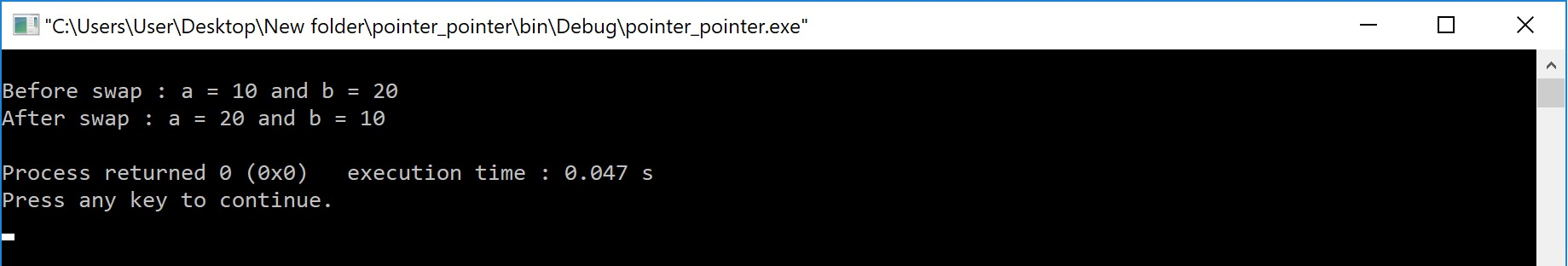The perfect place for easy learning...

×

# Pointers for Functions in C

In the c programming language, there are two ways to pass parameters to functions. They are as follows...

1. Call by Value
2. Call By Reference

We use pointer variables as formal parameters in call by reference parameter passing method.
In case of call by reference parameter passing method, the address of actual parameters is passed as arguments from the calling function to the called function. To recieve this address, we use pointer variables as formal parameters.

Consider the following program for swapping two variable values...

# Example - Swapping of two variable values using Call by Reference

``````#include<stdio.h>
#include<conio.h>

void swap(int *, int *) ;

void main()
{
int a = 10, b = 20 ;

clrscr() ;

printf(“Before swap : a = %d and b = %d\n", a, b) ;

swap(&a, &b) ;

printf(“After swap : a = %d and b = %d\n", a, b) ;

getch() ;
}
void swap(int *x, int *y)
{
int temp ;
temp = *x ;
*x = *y ;
*y = temp ;
}```
```

Output:In the above example program, we are passing the addresses of variables a and b and these are recieved by the pointer variables x and y. In the called function swap we use the pointer variables x and y to swap the values of variables a and b.